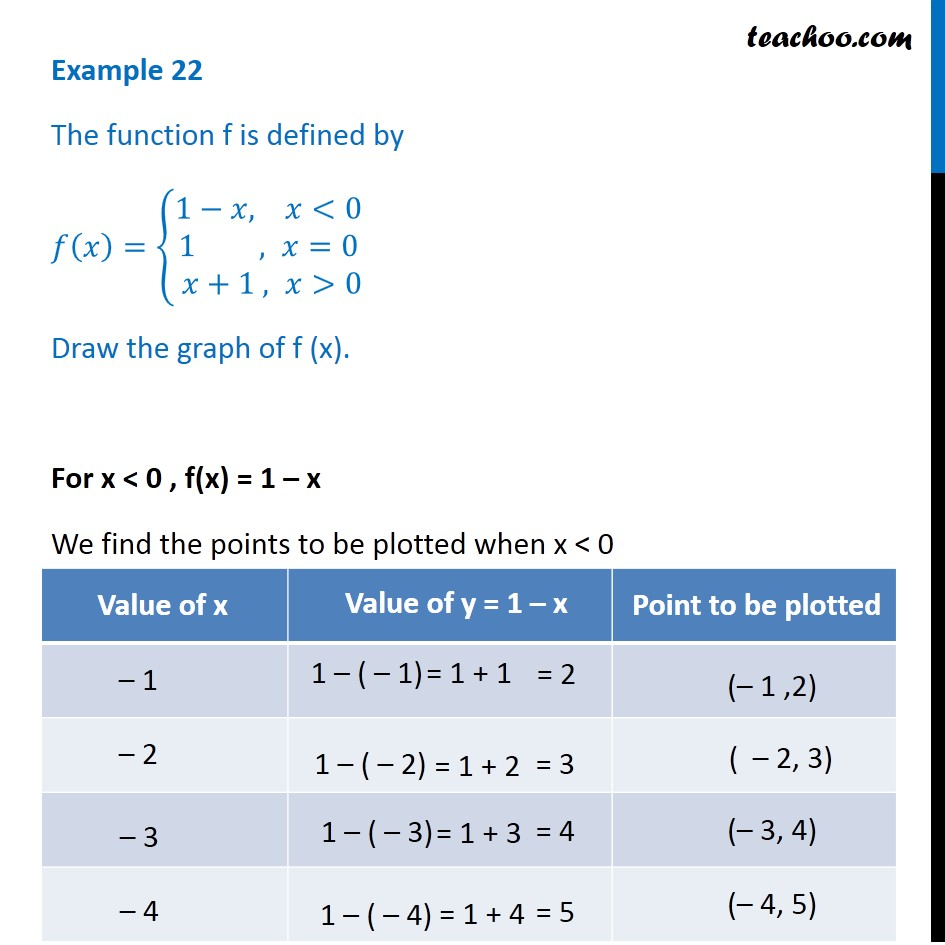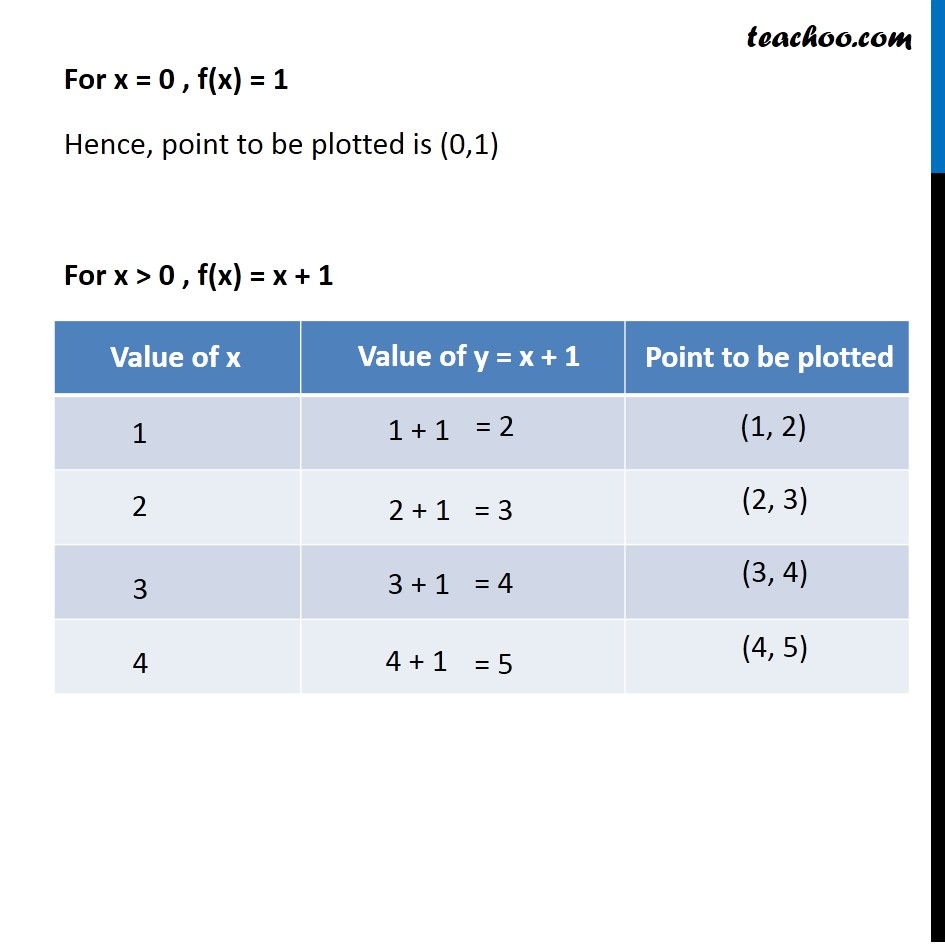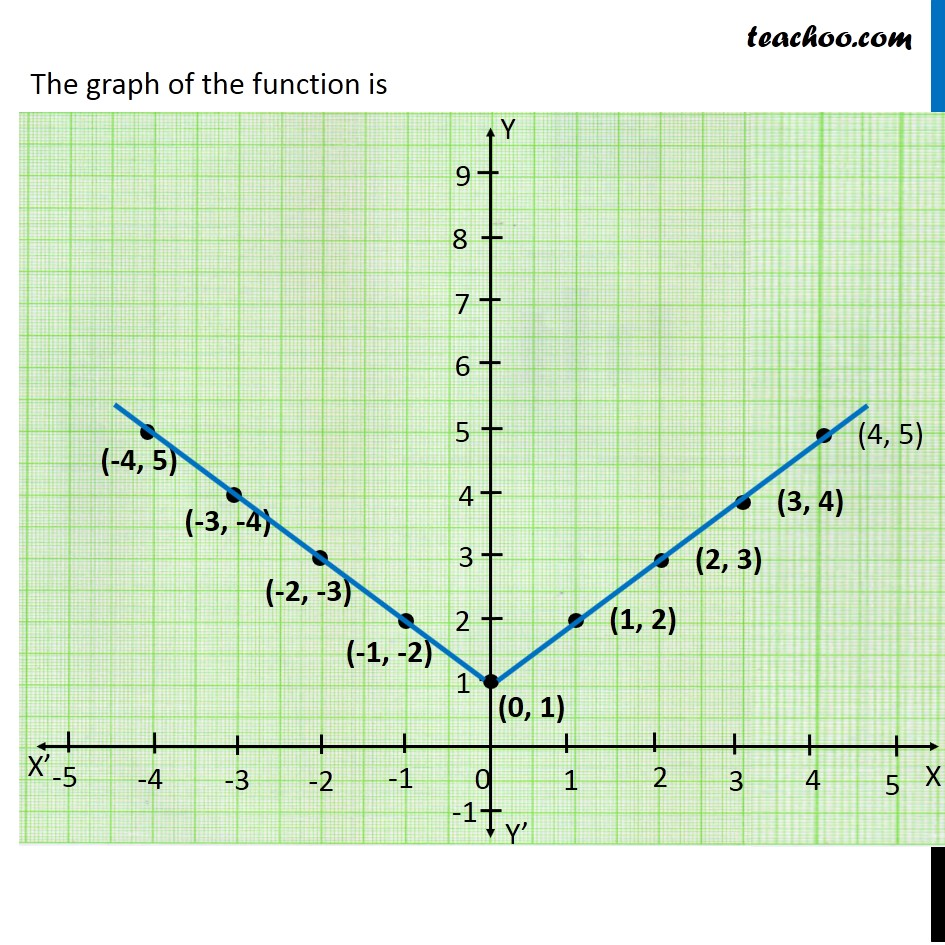Examples

Chapter 2 Class 11 Relations and Functions
Serial order wiseLearn in your speed, with individual attention - Teachoo Maths 1-on-1 Class

### Transcript

Example 22 The function f is defined by 𝑓(𝑥)={█(1−&𝑥, 𝑥<[email protected] , 𝑥=0@ 𝑥+1 , 𝑥>0)┤ Draw the graph of f (x). For x < 0 , f(x) = 1 – x We find the points to be plotted when x < 0 For x = 0 , f(x) = 1 Hence, point to be plotted is (0,1) For x > 0 , f(x) = x + 1 We find the points to be plotted when x > 0 Hence we make a graph The graph of the function is